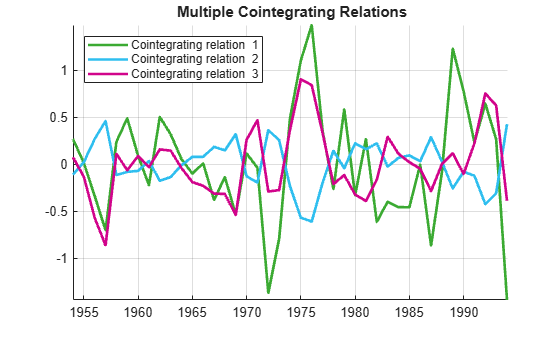Documentation

## Identifying Single Cointegrating Relations

### The Engle-Granger Test for Cointegration

Modern approaches to cointegration testing originated with Engle and Granger . Their method is simple to describe: regress the first component y1t of yt on the remaining components of yt and test the residuals for a unit root. The null hypothesis is that the series in yt are not cointegrated, so if the residual test fails to find evidence against the null of a unit root, the Engle-Granger test fails to find evidence that the estimated regression relation is cointegrating. Note that you can write the regression equation as ${y}_{1t}-{b}_{1}{y}_{2t}-...-{b}_{d}{y}_{dt}-{c}_{0}=\beta \prime {y}_{t}-{c}_{0}={\epsilon }_{t}$, where $\beta =\left[\begin{array}{cc}1& -b\prime \end{array}\right]\prime$ is the cointegrating vector and c0 is the intercept. A complication of the Engle-Granger approach is that the residual series is estimated rather than observed, so the standard asymptotic distributions of conventional unit root statistics do not apply. Augmented Dickey-Fuller tests (adftest) and Phillips-Perron tests (pptest) cannot be used directly. For accurate testing, distributions of the test statistics must be computed specifically for the Engle-Granger test.

The Engle-Granger test is implemented in Econometrics Toolbox™ by the function egcitest. For an example, see Test for Cointegration Using the Engle-Granger Test.

### Limitations of the Engle-Granger Test

The Engle-Granger method has several limitations. First of all, it identifies only a single cointegrating relation, among what might be many such relations. This requires one of the variables, ${y}_{1t}$, to be identified as "first" among the variables in ${y}_{t}$. This choice, which is usually arbitrary, affects both test results and model estimation. To see this, permute the three interest rates in the Canadian data and estimate the cointegrating relation for each choice of a "first" variable.

load Data_Canada Y = Data(:,3:end); % Interest rate data P0 = perms([1 2 3]); [~,idx] = unique(P0(:,1)); % Rows of P0 with unique regressand y1 P = P0(idx,:); % Unique regressions numPerms = size(P,1); % Preallocate: T0 = size(Y,1); H = zeros(1,numPerms); PVal = zeros(1,numPerms); CIR = zeros(T0,numPerms); % Run all tests: for i = 1:numPerms YPerm = Y(:,P(i,:)); [h,pValue,~,~,reg] = egcitest(YPerm,'test','t2'); H(i) = h; PVal(i) = pValue; c0i = reg.coeff(1); bi = reg.coeff(2:3); betai = [1;-bi] CIR(:,i) = YPerm*betai-c0i; end
betai = 3×1 1.0000 1.0718 -2.2209 
betai = 3×1 1.0000 -0.6029 -0.3472 
betai = 3×1 1.0000 -1.4394 0.4001 
 % Display the test results: H,PVal
H = 1×3 1 1 0 
PVal = 1×3 0.0202 0.0290 0.0625 

For this data, two regressands identify cointegration while the third regressand fails to do so. Asymptotic theory indicates that the test results will be identical in large samples, but the finite-sample properties of the test make it cumbersome to draw reliable inferences.

A plot of the identified cointegrating relations shows the previous estimate (Cointegrating relation 1), plus two others. There is no guarantee, in the context of Engle-Granger estimation, that the relations are independent: Plot the cointegrating relations:

h = gca; COrd = h.ColorOrder; h.NextPlot = 'ReplaceChildren'; h.ColorOrder = circshift(COrd,3); plot(dates,CIR,'LineWidth',2) title('{\bf Multiple Cointegrating Relations}') legend(strcat({'Cointegrating relation '}, ... num2str((1:numPerms)')),'location','NW'); axis tight grid onAnother limitation of the Engle-Granger method is that it is a two-step procedure, with one regression to estimate the residual series, and another regression to test for a unit root. Errors in the first estimation are necessarily carried into the second estimation. The estimated, rather than observed, residual series requires entirely new tables of critical values for standard unit root tests.

Finally, the Engle-Granger method estimates cointegrating relations independently of the VEC model in which they play a role. As a result, model estimation also becomes a two-step procedure. In particular, deterministic terms in the VEC model must be estimated conditionally, based on a predetermined estimate of the cointegrating vector. For an example of VEC model parameter estimation, see Estimate VEC Model Parameters Using egcitest.